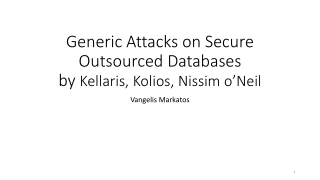Download PresentationGeneric Attacks on Secure Outsourced Databases by Kellaris , Kolios , Nissim o’Neil

# Generic Attacks on Secure Outsourced Databases by Kellaris , Kolios , Nissim o’Neil

Download Presentation## Generic Attacks on Secure Outsourced Databases by Kellaris , Kolios , Nissim o’Neil

- - - - - - - - - - - - - - - - - - - - - - - - - - - E N D - - - - - - - - - - - - - - - - - - - - - - - - - - -
##### Presentation Transcript

1. Generic Attacks on Secure Outsourced Databasesby Kellaris, Kolios, Nissimo’Neil Vangelis Markatos

2. What is the problem? • Users outsource their IT to the cloud • They store their data on the cloud • Because the cloud provides • 24/7 support • Fast access • very low cost. • Good! Now, WHAT IS THE PROBLEM? • The cloud provider can see all the data and find information • Banks– bank accounts of customers, balance, etc. • Hospitals – medical information of patients • Matchmaking services – who dates whom, etc.

3. Solution! • Encrypt all data on the cloud!!!! • GREAT! • The cloud provider can not see the data • Because they are encrypted • BUT… • How do does the client access them? • You can search encrypted data • E.g. SELECT * from TABLE where key = 0x32137183ABCFFDEE53621321

4. So, what is the problem now? • If the data are stored encrypted • If the data are searched encrypted • The cloud provider can not find any information. Right? • Right? • Right? • …. • …. • ….

5. Let us take a step back • Before we describe what the cloud provider can find • Let us describe what we have • We have a database:

6. Let us take a step back • We can ask queries to the database • SELECT * from TABLE where Age = 0x6576ABFFF7678888 • SELECT * from TABLE where • Age > 0x6576ABFFF7678888 AND Age < 0x5675867AFFBFDDEF67868987 • Range Queries • So: • Data are encrypted • Queries are encrypted • The user issues Range Queries

7. What is the problem? • The attacker tries to find information leaks • The attacker can not see the plaintext values • He observes the (encrypted) computation • Leaks: Assume that the attacker is able to see the • Volume of query result • Does the query return a lot of data? (Finding: probably large range) • SELECT * from TABLE where Age > 0 AND Age < 100 • Does the query return little data? (Finding: probably short rage query) • SELECT * from TABLE where Age > X AND Age < X+1

8. What is the problem? • Leaks: Assume that the attacker is able to see the • Access pattern of query result • SELECT * from TABLE where Age > 0x567856ABB AND Age < 0x5567877BDFAB • returns 0xABCB, 0XABBCD, 0x66789ABCDFFD • SELECT * from TABLE where Age > 0x43246ABB AND Age < 0x465467BDFAB • returns 0xABCB, 0XA6769BBCD • Finding: These queries must overlap!!

9. Attacker: Can we find the order of the elements? • By observing query results? • That return encrypted database elements? • Let us assume that we observe for enough time • So as to see all results of all queries • Let us assume that we know the minimum element (say 0xAB) • Find a query result that contains all elements • Find a query result that contains all element apart from 0xAB and one other one (say 0xAC) • That other one (0xAC) is the second smallest element

10. Can we find the order of the elements? • Find a query result that contains all element apart from 0xAB, 0xAC and one other one (say 0xAA) • That other one (0xAA) is the third smallest element • So the order is 0xAB, 0xAC, 0xAA, etc. • And so on and so forth… • After O(N) repetitions you will know the order of all elements

11. Find the order of the elements • Assume that the database contains elements • 1, 3, 5, 7, 9 • Assume that they are encrypted as • enc(1), enc(3), enc(5), enc(7), enc(9) • Assume that the attacker knows that enc(1) is the smallest element • Assume that the attacker has seen all query results. • These include the following query results • {enc(1), enc(3), enc(5), enc(7), enc(9)} • {enc(1)} and {enc(3), enc(5), enc(7), enc(9)} • {enc(1), enc(3} and {enc(5), enc(7), enc(9)} • {enc(1), enc(3), enc(5)} and {enc(7), enc(9)} • {enc(1), enc(3), enc(5), enc(7)} and {enc(9)}

12. Summary • The attacker found the order of the elements. • He knows that their order is • enc(1), enc(3), enc(5), enc(7), enc(9) • Although he does not know that “enc(1)” corresponds to “1”

13. Can we find the values that exist in the database? • i.e. we have found the order of the encrypted values of the elements • E.g. we have found that the elements and their order is: • enc(20,000) , enc(40,000), enc(70,000), and enc(80,000) • Can we find that the elements are: • 20,000 , 40,000, 70,000, and 80,000 ? • Assumption of the attacker: • The client issues queries uniformly at random • Note: any other query distribution would suit as well

14. This is our database • It is a salary database with points at • 20,000 , 40,000, 70,000, and 80,000 • The attacker sees query results that contain • enc(20,000) , enc(40,000), enc(70,000), and enc(80,000)

15. How many queries contain X results?

16. Reconstruct • Solve the equations to find r0, r1, r2, etc. r0=2, r1=2, r2=3, r3=1, r4=1

17. Summary • Data in the cloud stored encrypted • Database allows range queries • Attackers observes (encrypted) range query results • He is able to find • Order of database elements • Actual values of the records in the database…